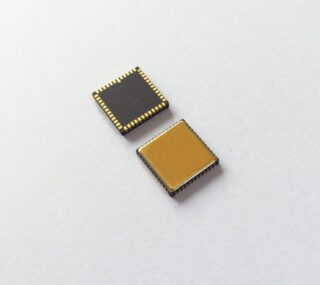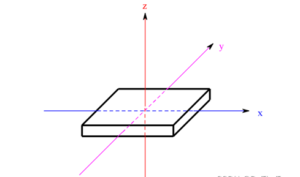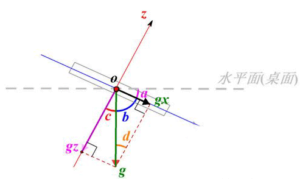Application

# Application of Six-axis IMU in Smart CarThe gyroscope is a sensor that senses the spatial attitude, and is the core element for controlling the balance of the car, judging and adjusting the attitude.

The six-axis IMU combines a three-axis gyroscope and a three-axis accelerometer. Its “six axes” are the acceleration xyz axis and the angular velocity xyz axis, which can sense both the angle change and the acceleration change. These six quantities are calculated , which can return three attitude angles:

Pitch angle (the tilt angle of the front of the car around the Y axis),

The heading angle (the rotation angle of the body around the Z axis),

Roll angle (the angle the side wheel lifts around the X axis).

1.The main functions of the gyroscope in the racing smart car are as follows:

It is used in cascade with the steering ring and is related to the Z-axis angular velocity to increase the steering force;

Adjust the posture of the balance car so that the body is balanced and can turn and move forward normally

The input of the angle loop closed-loop control, such as crossing the three-pronged road to make an angle of 60 degrees;

Determine the body posture and adjust the state. For example, I have sensed the angle change of the roundabout; sensed the rollover and protected it;

Temperature sensor, motor high temperature alarm (the gyroscope is generally close to the motor and has its own temperature sensor)

2.Basic usageA six-axis IMU combines a three-axis angular velocity and a three-axis accelerometer.

1.Angular Velocity Meter

The data returned by the angular velocity meter is actually the angular velocity in radians. When the gyroscope is rotated, the angular velocity reference value of the corresponding axis is returned. Generally, we need to integrate the angular velocity to obtain the angle, which is intuitive and convenient to use. The angle obtained by the integration is the relative angle, and the attitude at the beginning of the integration is the reference 0 angle.

The integral value of 2ms is about 0.000124, which needs to be adjusted in actual use. Rotate the gyroscope once, and the calculated angle should be 360.

Integrating always introduces error, which increases over time. In addition, the gyroscope also has a zero drift phenomenon, that is, when the car is stationary, it will output a non-zero angular velocity value. The offset value needs to be recalibrated every time the power is turned on, or it needs to be calibrated regularly. Subsequent detection can be eliminated by subtracting the offset value. this error.

The acceleration returned by the accelerometer is not an acceleration in the general sense. The accelerometer is equivalent to a spring system with a weight in the middle and springs in all directions supporting it. When laying flat on the table, some springs are stretched and some are flattened. When changing, different springs are compressed differently, thereby detecting forces in different directions. So actually standing still also produces an “acceleration” value due to gravity. Note that this “acceleration” is not stable, because the acceleration generated by the swing of the car model itself will generate a large interference signal, so it is not appropriate to rely too much on this value.

Only the accelerometer can be used to get the pitch angle of the body, and it is the absolute angle to the ground. In the swing accelerometer, the gravitational acceleration component of the corresponding axis changes, and the change of the angle can be obtained by using the inverse trigonometric function. However, due to the instability of the accelerometer, superimposed on the gravity measurement signal, the output signal cannot reflect the inclination of the car model well.3.More accurate data processing

Because the data has errors, instability, and zero drift, the data needs to be processed.

1.Filtering

The input stage can use arithmetic average filtering, and it is generally stable to take the average of ten times. If you want to use acceleration, you can add a low-pass filter with a relatively small coefficient.

Remember to remove the zero offset value from the angular velocity.

2.Angle fusion

The angle obtained by the angular velocity integration is relatively stable, but it will drift slowly over time and only reflects the amount of change; the angle obtained by the inverse trigonometric function of the acceleration is unstable, but it follows the real angle and is an absolute angle to the ground.

The angle variable is used in the whole process of the balance car. The drift deviation caused by the simple angular velocity integration cannot be ignored, and the reference point is not easy to determine. Therefore, it is necessary to use angle fusion to filter and fuse the angles obtained from the two. A more reliable angle can be obtained.

The most commonly used methods of angle fusion filtering are: complementary filtering, second-order complementary filtering, Kalman filtering, Tsinghua filtering, and quaternion filtering.

The last two statements are the most critical part, so a brief explanation:

Current value (gyroscope) = last value + angular velocity differential value

Current value (fusion) = current value (gyroscope) * (1-a) – current value (acceleration) * a (a is the weight)

The angle obtained by the gyroscope integration is stable, and the angle obtained by the acceleration is the closest to the true value.

Whether it is complementary filtering or quaternion filtering, you need to re-adjust the parameters after getting them, and the parameters are different for different cars.

When the car starts to start, it should be noted that if the angle value is obtained by the fusion algorithm, there will be a process of gradually transitioning from the angular velocity integral with a relatively small value to the real value, and the duration is about 0.3s to 1s, so this period of time just started It is not appropriate to use the fusion angle, and the angle obtained by inverting the trigonometric function with only the acceleration is more realistic.

Six-axis Gyroscope in Smart Car

mems gyro manufacturers

mems gyro

gyro imu

gyro technology

gyro market